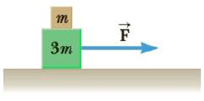Chapter 4, Problem 68P

Chapter
Section
Textbook Problem

A block of mass 3m is placed on a frictionless horizontal surface, and a second block of mass m is placed on top of the first block. The surfaces of the blocks are rough. A constant force of magnitude F is applied to the first block as in Figure P4.68. (a) Construct free-body diagrams for each block. (b) Identify the horizontal force that causes the block of mass m to accelerate. (c) Assume that the upper block does not slip on the lower block, and find the acceleration of each block in terms of m and F.Figure P4.68

(a)

To determine
The free body diagram of each block.

Explanation

Given info: The masses are m and 3m. The constant horizontal force is F.

The free body diagram for the upper block.

• N1 is the normal force.
• Fs is the static friction force.
• g is the acceleration due to gravity.

The free body diagram for the lower block

(b)

To determine
The force causing the upper block to move.

(c)

To determine
The acceleration of the blocks.

Still sussing out bartleby?

Check out a sample textbook solution.

See a sample solution

The Solution to Your Study Problems

Bartleby provides explanations to thousands of textbook problems written by our experts, many with advanced degrees!

Get Started